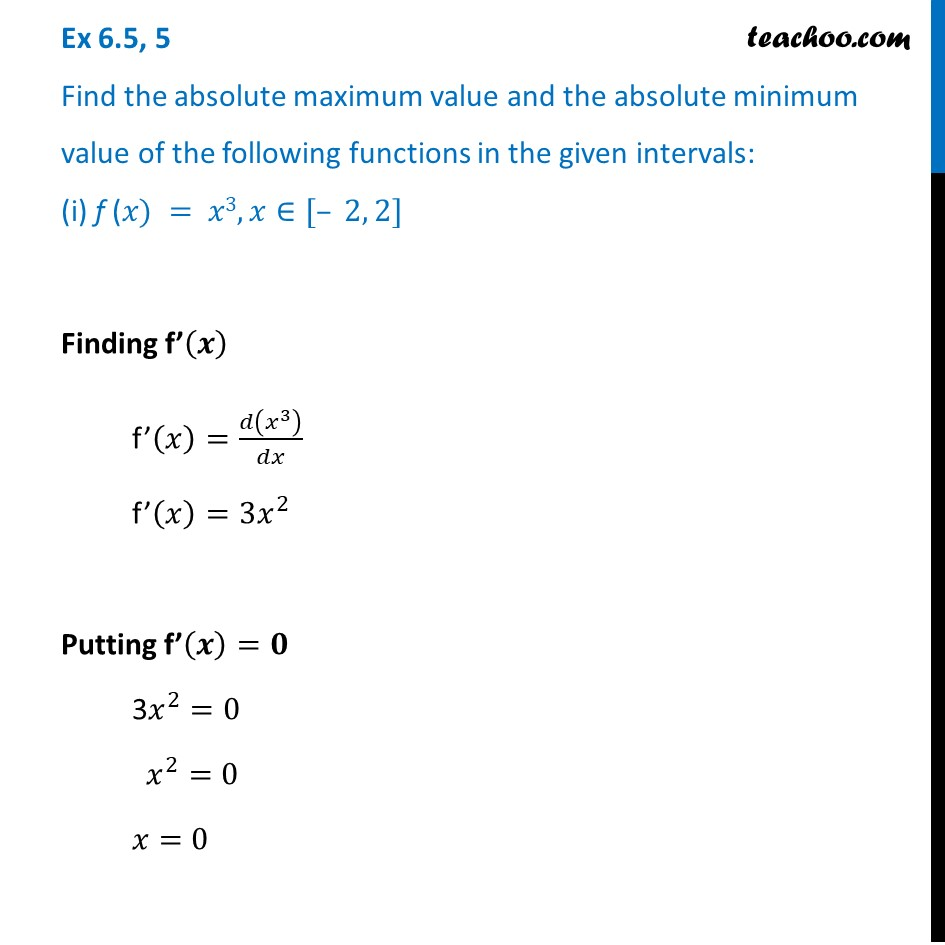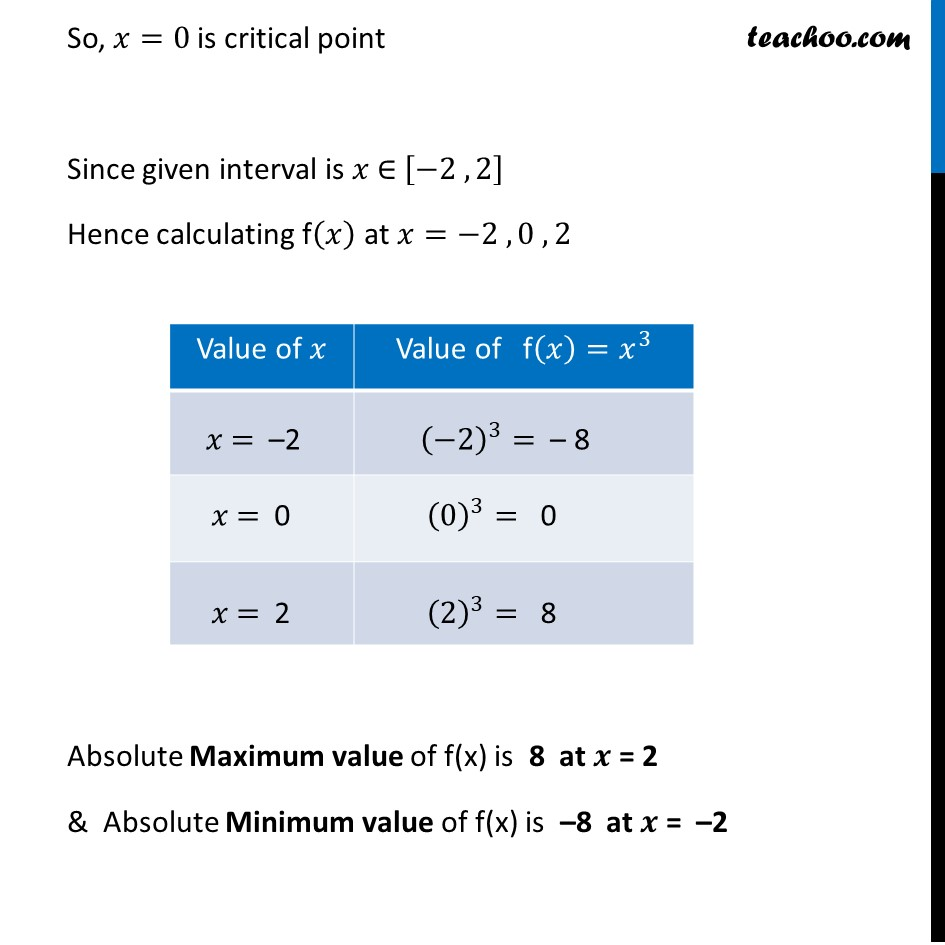Absolute minima/maxima

Chapter 6 Class 12 Application of Derivatives (Term 1)
Concept wise### Transcript

Ex 6.5, 5 Find the absolute maximum value and the absolute minimum value of the following functions in the given intervals: (i) f (𝑥) = 𝑥3, 𝑥∈[– 2, 2]Finding f’(𝒙) f’(𝑥)=𝑑(𝑥^3 )/𝑑𝑥 f’(𝑥)=3𝑥^2 Putting f’(𝒙)=𝟎 3𝑥^2=0 𝑥^2=0 𝑥=0 So, 𝑥=0 is critical point Since given interval is 𝑥 ∈ [−2 , 2] Hence calculating f(𝑥) at 𝑥=−2 , 0 , 2 Absolute Maximum value of f(x) is 8 at 𝒙 = 2 & Absolute Minimum value of f(x) is –8 at 𝒙 = –2